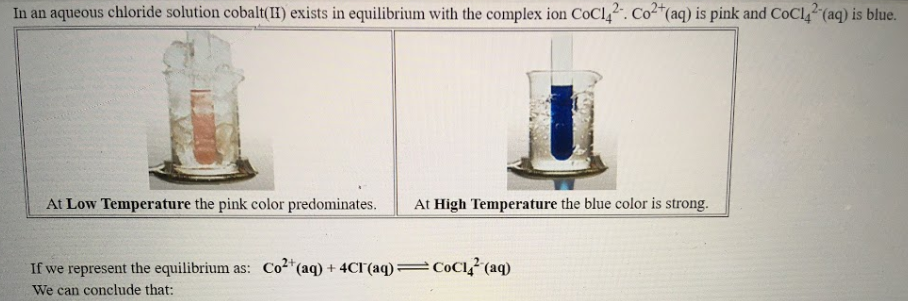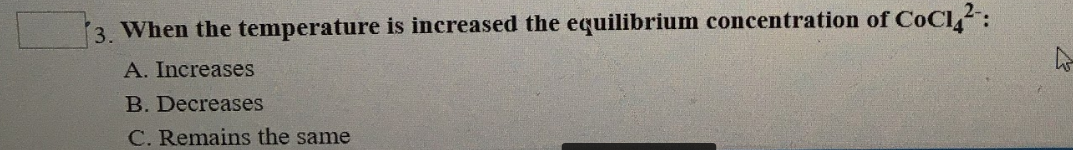# In an aqueous chloride solution cobalt (II) exists in equilibrium with the complex ion CoCl4^2-• Co^2+ (aq) is pink and CoCl4^2-(aq) is blue. If we represent the equilibrium as: CO^2+(aq) + 4Cl^-(aq) ⇌ CoCl4^2- (aq) We can conclude that: 3. When the temperature is increased the equilibrium concentration of CoCl4^2-: A. Increases B. Decreases C. Remains the same